Life In 19x19http://lifein19x19.com/ seki and dying: a fine linehttp://lifein19x19.com/viewtopic.php?f=4&t=16455 Page 1 of 1

 Author: Ian Butler [ Sun Feb 17, 2019 10:43 am ] Post subject: seki and dying: a fine line So with Spring in the air and me having taken a trip down memory lane, I really wanted to play a game. I'm sick of being concerned with improving and whatnot. I decided to just play.It's online, but I try not to blitz. Today it worked out okay at some points, though I completely ceased thinking and reading on the bottom, mindlessly imitating plays I remember from another context.So while I got ahead initially, I lost my lead and was behind by 30 points there. Quite a lapse in concentration.I do something desperate and it only takes two mistakes by my opponent to swing the game back to my favour. One is him missing a chance at seki instead of handing me the kill.What follows is a great test (for someone like me, who is very prone to give away games where I'm leading, losing focus especially on computer Go) to stay focused and stay strong. Today I managed fine and black couldn't catch me with my pants down again.Result:Bad: I still played relatively blitz-yBad: the bottom sequence. I can't even ... Terrible ... Just noGood: I find my focus again to go for the kill and to fend off Black's further attacks, both on the left and in the right bottom corner.Good: Attack with a plan and by gaining territory (a lot of it)I want to take home one or two lessons from each game. So I hope to get some help deciding what lessons to learn from this one. I think one is:- don't push your opponent to your own weak group (I attacked to make profit, but in turn weakened my own group. In my defense, I thought it was alive with 2 eyes. It almost was, but in the end it almost died / sekiedMaybe for the 2nd lesson:- Connect back up if you can instead of letting yourself getting surrounded without certainty of life.What do you think? Good conclusions based on the game?Thanks! Some self review with Leela, comments in the file. Stops at move 164, except for a final comment at the end, about the corner up top.

 Author: Joaz Banbeck [ Mon Feb 18, 2019 2:01 pm ] Post subject: Re: seki and dying: a fine line Click Here To Show Diagram Code`[go]\$\$ The F5 stone is too high. Undercut it while pincering.\$\$ ---------------------------------------\$\$ | . . . . X . . . . . . . . . . . . . . |\$\$ | . . . . . X O . . . . . . . . . . . . |\$\$ | . . X X X O . O . . . . . . . X . . . |\$\$ | X X O O O O . . . , . . . . . , . . . |\$\$ | . O . . . . . . . . . . . . . . X . . |\$\$ | . . . . . . . . . . . . . . . . . . . |\$\$ | . . . . . . . . . . . . . . . . . . . |\$\$ | . . . . . . . . . . . . . . . . . . . |\$\$ | . . . . . . . . . . . . . . . . . . . |\$\$ | . . . O . . . . . , . . . . . , . . . |\$\$ | . . . . . . . . . . . . . . . . . . . |\$\$ | . . . . . . . . . . . . . . . . . . . |\$\$ | . . . . . . . . . . . . . . . . . . . |\$\$ | . . O O . . . . . . . . . . . . . . . |\$\$ | . . X . . X . . . . . . . . . . . . . |\$\$ | . . . X . . . . . 2 . . . . . O . . . |\$\$ | . . . . . . . . . . . . . 1 . . . . . |\$\$ | . . . . . . . . . . . . . . . . . . . |\$\$ | . . . . . . . . . . . . . . . . . . . |\$\$ ---------------------------------------[/go]` Click Here To Show Diagram Code`[go]\$\$ Use that strength on the left\$\$ ---------------------------------------\$\$ | . . . . X . . . . . . . . . . . . . . |\$\$ | . . . . . X O . . . . . . . . . . . . |\$\$ | . . X X X O . O . . . O . . . X . . . |\$\$ | X X O O O O . . . 1 . . . . . , . . . |\$\$ | . O . . . . . . . . 2 . . . . . X . . |\$\$ | . . . . . . . . . . . . . . . . . . . |\$\$ | . . . . . . . . . . . . . . . . . . . |\$\$ | . . . . . . . . . . . . . . . . . . . |\$\$ | . . . . . . . . . . . . . . . . . . . |\$\$ | . . . O . . . . . , . . . . . , . . . |\$\$ | . . . . . . . . . . . . . . . . . . . |\$\$ | . . . . . . . . . . . . . . . . . . . |\$\$ | . . . . . . . . . . . . . . . . . . . |\$\$ | . . O O . . . . . . . . . . . . O . . |\$\$ | . . X . . X . . . . . . . . . . . . . |\$\$ | . . . X . . . . . , X . . . . O . . . |\$\$ | . . . . . . . . . . . . . X . . . . . |\$\$ | . . . . . . . . . . . . . . . . . . . |\$\$ | . . . . . . . . . . . . . . . . . . . |\$\$ ---------------------------------------[/go]` Click Here To Show Diagram Code`[go]\$\$ Position at move 35\$\$ ---------------------------------------\$\$ | . . . . X . . . . . . . . . . . . . . |\$\$ | . . . . . X O . . . . . . . . . . . . |\$\$ | . . X X X O . O . O O O 1 3 . X . . . |\$\$ | X X O O O O . . . X X X O . . , . . . |\$\$ | . O . . . . . . . . . . 2 . . . X . . |\$\$ | . . . . . . . . . . 4 . . . . . . . . |\$\$ | . . . . . . . . . . . . . . . . . . . |\$\$ | . . . . . . . . . . . . . . . . . . . |\$\$ | . . . . . . . . . . . . . . . . . . . |\$\$ | . . . O . . . . . , . . . . . , . . . |\$\$ | . . . . . . . . . . . . . . . . . . . |\$\$ | . . . . . . . . . . . . . . . . . . . |\$\$ | . . . . . . . . . . . . . . . . . . . |\$\$ | . . O O . . . . . . . . . . . . O . . |\$\$ | . . X . . X . . . . . . . . . . . . . |\$\$ | . . . X . . . . . , X . . . . O . . . |\$\$ | . . . . . . . . . . . . . X . . . . . |\$\$ | . . . . . . . . . . . . . . . . . . . |\$\$ | . . . . . . . . . . . . . . . . . . . |\$\$ ---------------------------------------[/go]` Click Here To Show Diagram Code`[go]\$\$ This is the vital point\$\$ ---------------------------------------\$\$ | . . . . X . . . . . . . . . . . . . . |\$\$ | . . . . . X O . . . . . . . . . . . . |\$\$ | . . X X X O . O . O O O X X X X . . . |\$\$ | X X O O O O . . . X X X O O O , . . . |\$\$ | . O . . . . . . . . . . . . . . X . . |\$\$ | . . . . . . . . . . . . . . . . . . . |\$\$ | . . . . . . . . . . . X . O . O X . . |\$\$ | . . . . . . . . . . . . . . O X O . . |\$\$ | . . . . . . . . . . . . . O . 1 . . . |\$\$ | . . . O . . . . . , . . . O X X X 2 . |\$\$ | . . . . . . . . . . . . . X O O O . . |\$\$ | . . . . . . . . . . . . . . X X O . . |\$\$ | . . . . . . . . . . . . . . . . . . . |\$\$ | . . O O . . . . . . . . . . . . O . . |\$\$ | . . X . . X . . . . . . . . . . . . . |\$\$ | . . . X . . . . . , X . . . . O . . . |\$\$ | . . . . . . . . . . . . . X . . . . . |\$\$ | . . . . . . . . . . . . . . . . . . . |\$\$ | . . . . . . . . . . . . . . . . . . . |\$\$ ---------------------------------------[/go]` Click Here To Show Diagram Code`[go]\$\$ So big that it is worth repeating: play here\$\$ ---------------------------------------\$\$ | . . . . X . . . . . . . . . . . . . . |\$\$ | . . . . . X O . . . . . . . . . . . . |\$\$ | . . X X X O . O . O O O X X X X . . . |\$\$ | X X O O O O . . . X X X O O O , 1 . . |\$\$ | . O . . . . . . . . . . . . . O X . . |\$\$ | . . . . . . . . . . . . . . . O O X . |\$\$ | . . . . . . . . . . . X . O . O X X . |\$\$ | . . . . . . . . . . . . . . O X O . . |\$\$ | . . . . . . . . . . . . . O . X . . . |\$\$ | . . . O . . . . . , . . . O X X X 2 . |\$\$ | . . . . . . . . . . . . . X O O O . . |\$\$ | . . . . . . . . . . . . . . X X O . . |\$\$ | . . . . . . . . . . . . . . . . . . . |\$\$ | . . O O . . . . . . . . . . . . O . . |\$\$ | . . X . . X . . . . . . . . . . . . . |\$\$ | . . . X . . . . . , X . . . . O . . . |\$\$ | . . . . . . . . . . . . . X . . . . . |\$\$ | . . . . . . . . . . . . . . . . . . . |\$\$ | . . . . . . . . . . . . . . . . . . . |\$\$ ---------------------------------------[/go]` Click Here To Show Diagram Code`[go]\$\$W If you want to attack, get the vital point, and force him toward your strength\$\$ ---------------------------------------\$\$ | . . . . X . . . . . . . . . . . . . . |\$\$ | . . . . . X O . . . . . . . . . . . . |\$\$ | . . X X X O . O . O O O X X X X . . . |\$\$ | X X O O O O . . . X X X O O O , X . . |\$\$ | . O . . . . . . . . . . . . . O X . . |\$\$ | . . . . . . . . . . 1 . . . . O O X . |\$\$ | . . . . . . . . . . . X . O . O X X . |\$\$ | . . . . . . . . . . . . . . O X O . . |\$\$ | . . . . . . . . . . . . . O . X . . . |\$\$ | . . . O . . . . . , . O . O X X X X . |\$\$ | . . . . . . . . . . . . O X O O O . . |\$\$ | . . . . . . . . . . . . O X X X O . . |\$\$ | . . . . . . . . . . . O X X . . . . . |\$\$ | . . O O . . . . . . . . O X . . O . . |\$\$ | . . X . . X . . . . . . . . . . . . . |\$\$ | . . . X . . . . . , X . . . . O . . . |\$\$ | . . . . . . . . . . . . . X . . . . . |\$\$ | . . . . . . . . . . . . . . . . . . . |\$\$ | . . . . . . . . . . . . . . . . . . . |\$\$ ---------------------------------------[/go]` Click Here To Show Diagram Code`[go]\$\$W However, this may be bigger.\$\$ ---------------------------------------\$\$ | . . . . X . . . . . . . . . . . . . . |\$\$ | . . . . . X O . . . . . . . . . . . . |\$\$ | . . X X X O . O . O O O X X X X . . . |\$\$ | X X O O O O . . . X X X O O O , X . . |\$\$ | . O . . . . . . . . . . . . . O X . . |\$\$ | . . . . . . . . . . . . . . . O O X . |\$\$ | . . . . . . . . . . . X . O . O X X . |\$\$ | . . . . . . . . . . . . . . O X O . . |\$\$ | . . . . . . . . . . . . . O . X . . . |\$\$ | . . . O . . . . . , . O . O X X X X . |\$\$ | . . . . . . . . . . . . O X O O O . . |\$\$ | . . . . . . . . . . . . O X X X O . . |\$\$ | . . . . . . . . . . . O X X . . . . . |\$\$ | . . O O . . . . . . . . O X . . O . . |\$\$ | . . X . . X . . . . . . . 1 . . . . . |\$\$ | . . . X . . . . . , X . . . . O . . . |\$\$ | . . . . . . . . . . . . . X . . . . . |\$\$ | . . . . . . . . . . . . . . . . . . . |\$\$ | . . . . . . . . . . . . . . . . . . . |\$\$ ---------------------------------------[/go]`Two concepts to think about to improve your game:Note in the fourth and seventh diagrams, I suggest plays that probably won't kill, but will saddle your opponent with weak groups. Sometimes the burden of continually defending those groups may be worse than losing them.Also, check https://senseis.xmp.net/?VitalPoint. Try to grasp the essence of the concept of a vital point.

 Author: Knotwilg [ Mon Feb 18, 2019 4:05 pm ] Post subject: Re: seki and dying: a fine line At 237 what if Black D7? I had to look at it a few times.

 Author: Joaz Banbeck [ Mon Feb 18, 2019 7:07 pm ] Post subject: Re: seki and dying: a fine line Knotwilg wrote:At 237 what if Black D7? I had to look at it a few times.It is tricky. I think A10 defends.

 Author: Bill Spight [ Mon Feb 18, 2019 10:56 pm ] Post subject: Re: seki and dying: a fine line Joaz Banbeck wrote:Knotwilg wrote:At 237 what if Black D7? I had to look at it a few times.It is tricky. I think A10 defends.Then White can run out, right?Author: Bill Spight [ Mon Feb 18, 2019 11:01 pm ] Post subject: Re: seki and dying: a fine line Several comments with some tricky variations. No guarantee I got everything right, OC.Main foci: 1) As a rule, do not attack from behind. Get ahead or in front.2) Don't go after the small stuff. Not at first, anyway. 3) Attack potential eyes, as a rule.4) Keima for attack.

 Author: Joaz Banbeck [ Mon Feb 18, 2019 11:50 pm ] Post subject: Re: seki and dying: a fine line Joaz Banbeck wrote:Knotwilg wrote:At 237 what if Black D7? I had to look at it a few times.It is tricky. I think A10 defends.Although with black's best play, white has to give up A5 and B5, but he escapes northward. Click Here To Show Diagram Code`[go]\$\$ Position at move 237\$\$ ---------------------------------------\$\$ | . . . . X . . . . . . . . . . . . . . |\$\$ | . X . . . X O . . . . O O X . . . . . |\$\$ | . . X X X O . O . O O O X X X X . . . |\$\$ | X X O O O O . . O X X X O O O X X . . |\$\$ | O O . . . . . . . O . X X O O O X . . |\$\$ | . . . O . . . O O X X X X X . O O X . |\$\$ | . . O O O X X O X X O X O O O O X X . |\$\$ | . 8 O . O O X O X . O X X X O X O . . |\$\$ | 6 X X O X O O X X X O O X O O X . . . |\$\$ | 2 O X , X X O X O X X O O O X X X X . |\$\$ | . O X X . O . O O X O . O X O O O O X |\$\$ | 3 O O X X O O O X O O O O X X X O X X |\$\$ | 5 O 4 1 O X X X X X X O X X . . O X . |\$\$ | . 7 O O O O O O X X . X . X . . O O . |\$\$ | O O X X O X X X . X . X . X O O . . . |\$\$ | X X . X X . . O . , X . X X X O . O O |\$\$ | . . . . . . X . . X . . . X X O O X O |\$\$ | . . . . . . . O . . X . X X O O X . X |\$\$ | . . . . . . . . . . . X X O . O . X . |\$\$ ---------------------------------------[/go]`Trying to save those two stones is not good for white. Click Here To Show Diagram Code`[go]\$\$\$\$ ---------------------------------------\$\$ | . . . . X . . . . . . . . . . . . . . |\$\$ | . X . . . X O . . . . O O X . . . . . |\$\$ | . . X X X O . O . O O O X X X X . . . |\$\$ | X X O O O O . . O X X X O O O X X . . |\$\$ | O O . . . . . . . O . X X O O O X . . |\$\$ | . . . O . . . O O X X X X X . O O X . |\$\$ | . . O O O X X O X X O X O O O O X X . |\$\$ | 9 . O . O O X O X . O X X X O X O . . |\$\$ | 8 X X O X O O X X X O O X O O X . . . |\$\$ | 2 O X , X X O X O X X O O O X X X X . |\$\$ | . O X X . O . O O X O . O X O O O O X |\$\$ | 3 O O X X O O O X O O O O X X X O X X |\$\$ | 4 O 6 1 O X X X X X X O X X . . O X . |\$\$ | 7 5 O O O O O O X X . X . X . . O O . |\$\$ | O O X X O X X X . X . X . X O O . . . |\$\$ | X X . X X . . O . , X . X X X O . O O |\$\$ | . . . . . . X . . X . . . X X O O X O |\$\$ | . . . . . . . O . . X . X X O O X . X |\$\$ | . . . . . . . . . . . X X O . O . X . |\$\$ ---------------------------------------[/go]`How many of us would see A8 over the board?

 Author: Bill Spight [ Tue Feb 19, 2019 12:09 am ] Post subject: Re: seki and dying: a fine line Click Here To Show Diagram Code`[go]\$\$ Position at move 237\$\$ ---------------------------------------\$\$ | . . . . X . . . . . . . . . . . . . . |\$\$ | . X . . . X O . . . . O O X . . . . . |\$\$ | . . X X X O . O . O O O X X X X . . . |\$\$ | X X O O O O . . O X X X O O O X X . . |\$\$ | O O . . . . . . . O . X X O O O X . . |\$\$ | . . . O . . . O O X X X X X . O O X . |\$\$ | . . O O O X X O X X O X O O O O X X . |\$\$ | . . O . O O X O X . O X X X O X O . . |\$\$ | 6 X X O X O O X X X O O X O O X . . . |\$\$ | 2 O X , X X O X O X X O O O X X X X . |\$\$ | . O X X . O . O O X O . O X O O O O X |\$\$ | 3 O O X X O O O X O O O O X X X O X X |\$\$ | 5 O . 1 O X X X X X X O X X . . O X . |\$\$ | . 4 O O O O O O X X . X . X . . O O . |\$\$ | O O X X O X X X . X . X . X O O . . . |\$\$ | X X . X X . . O . , X . X X X O . O O |\$\$ | . . . . . . X . . X . . . X X O O X O |\$\$ | . . . . . . . O . . X . X X O O X . X |\$\$ | . . . . . . . . . . . X X O . O . X . |\$\$ ---------------------------------------[/go]` Click Here To Show Diagram Code`[go]\$\$ Position at move 237\$\$ ---------------------------------------\$\$ | . . . . X . . . . . . . . . . . . . . |\$\$ | . X . . . X O . . . . O O X . . . . . |\$\$ | . . X X X O . O . O O O X X X X . . . |\$\$ | X X O O O O . . O X X X O O O X X . . |\$\$ | O O . . . . . . . O . X X O O O X . . |\$\$ | . 9 . O . . . O O X X X X X . O O X . |\$\$ | . . O O O X X O X X O X O O O O X X . |\$\$ | . . O . O O X O X . O X X X O X O . . |\$\$ | 8 X X O X O O X X X O O X O O X . . . |\$\$ | 2 O X , X X O X O X X O O O X X X X . |\$\$ | . O X X . O . O O X O . O X O O O O X |\$\$ | 7 O O X X O O O X O O O O X X X O X X |\$\$ | . O 5 1 O X X X X X X O X X . . O X . |\$\$ | 4 3 O O O O O O X X . X . X . . O O . |\$\$ | O O X X O X X X . X . X . X O O . . . |\$\$ | X X . X X . . O . , X . X X X O . O O |\$\$ | . . . . . . X . . X . . . X X O O X O |\$\$ | . . . . . . . O . . X . X X O O X . X |\$\$ | . . . . . . . . . . . X X O . O . X . |\$\$ ---------------------------------------[/go]`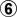@ 3.Edit: Oh!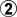doesn't work, does it? White has to lose some stones.

 Author: Bill Spight [ Tue Feb 19, 2019 1:55 am ] Post subject: Re: seki and dying: a fine line Click Here To Show Diagram Code`[go]\$\$c Seki\$\$ ---------------------------------------\$\$ | . . . . X . . . . . . . . . . . . . . |\$\$ | . X . . . X O . . . . O O X . . . . . |\$\$ | . . X X X O . O . O O O X X X X . . . |\$\$ | X X O O O O . . O X X X O O O X X . . |\$\$ | O O . . . . . . . O . X X O O O X . . |\$\$ | . . . O . . . O O X X X X X . O O X . |\$\$ | . . O O O X X O X X O X O O O O X X . |\$\$ | 4 6 O 8 O O X O X . O X X X O X O . . |\$\$ | 5 X X O X O O X X X O O X O O X . . . |\$\$ | 3 O X 0 X X O X O X X O O O X X X X . |\$\$ | 7 O X X b O . O O X O . O X O O O O X |\$\$ | 9 O O X X O O O X O O O O X X X O X X |\$\$ | a O . 1 O X X X X X X O X X . . O X . |\$\$ | . 2 O O O O O O X X . X . X . . O O . |\$\$ | O O X X O X X X . X . X . X O O . . . |\$\$ | X X . X X . . O . , X . X X X O . O O |\$\$ | . . . . . . X . . X . . . X X O O X O |\$\$ | . . . . . . . O . . X . X X O O X . X |\$\$ | . . . . . . . . . . . X X O . O . X . |\$\$ ---------------------------------------[/go]`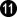@ a ,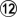@ b Click Here To Show Diagram Code`[go]\$\$c Ko\$\$ ---------------------------------------\$\$ | . . . . X . . . . . . . . . . . . . . |\$\$ | . X . . . X O . . . . O O X . . . . . |\$\$ | . . X X X O . O . O O O X X X X . . . |\$\$ | X X O O O O . . O X X X O O O X X . . |\$\$ | O O . . . . . . . O . X X O O O X . . |\$\$ | . . . O . . . O O X X X X X . O O X . |\$\$ | . 8 O O O X X O X X O X O O O O X X . |\$\$ | 9 7 O 0 O O X O X . O X X X O X O . . |\$\$ | 6 X X O X O O X X X O O X O O X . . . |\$\$ | 3 O X , X X O X O X X O O O X X X X . |\$\$ | 4 O X X . O . O O X O . O X O O O O X |\$\$ | . O O X X O O O X O O O O X X X O X X |\$\$ | 5 O . 1 O X X X X X X O X X . . O X . |\$\$ | . 2 O O O O O O X X . X . X . . O O . |\$\$ | O O X X O X X X . X . X . X O O . . . |\$\$ | X X . X X . . O . , X . X X X O . O O |\$\$ | . . . . . . X . . X . . . X X O O X O |\$\$ | . . . . . . . O . . X . X X O O X . X |\$\$ | . . . . . . . . . . . X X O . O . X . |\$\$ ---------------------------------------[/go]` Click Here To Show Diagram Code`[go]\$\$cm11 Ko\$\$ ---------------------------------------\$\$ | . . . . X . . . . . . . . . . . . . . |\$\$ | . X . . . X O . . . . O O X . . . . . |\$\$ | . . X X X O . O . O O O X X X X . . . |\$\$ | X X O O O O . . O X X X O O O X X . . |\$\$ | O O . . . . . . . O . X X O O O X . . |\$\$ | . . . O . . . O O X X X X X . O O X . |\$\$ | . O O O O X X O X X O X O O O O X X . |\$\$ | X X O O O O X O X . O X X X O X O . . |\$\$ | O X X O X O O X X X O O X O O X . . . |\$\$ | 5 O X 2 X X O X O X X O O O X X X X . |\$\$ | O O X X 4 O . O O X O . O X O O O O X |\$\$ | 6 O O X X O O O X O O O O X X X O X X |\$\$ | X O 1 X O X X X X X X O X X . . O X . |\$\$ | 3 O O O O O O O X X . X . X . . O O . |\$\$ | O O X X O X X X . X . X . X O O . . . |\$\$ | X X . X X . . O . , X . X X X O . O O |\$\$ | . . . . . . X . . X . . . X X O O X O |\$\$ | . . . . . . . O . . X . X X O O X . X |\$\$ | . . . . . . . . . . . X X O . O . X . |\$\$ ---------------------------------------[/go]`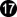throw in at 13,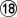= koWhere is a good ko threat for Black?G-19, huh?

 Author: Bill Spight [ Tue Feb 19, 2019 12:27 pm ] Post subject: Re: seki and dying: a fine line And the root of the problem,, seems to be move 204. Click Here To Show Diagram Code`[go]\$\$W Move 204\$\$ ---------------------------------------\$\$ | . . . . X . . . . . . . . . . . . . . |\$\$ | . X . . . X O . . . . . . . . . . . . |\$\$ | . . X X X O . O . O O O X X X X . . . |\$\$ | X X O O O O . . O X X X O O O X X . . |\$\$ | O O . . . . . . . O . X X O O O X . . |\$\$ | . . . O . . . O O X X X X X . O O X . |\$\$ | . . O O O X X O X X O X O O O O X X . |\$\$ | . . O . O O X O X . O X X X O X O . . |\$\$ | . X X O X O O X X X O O X O O X . . . |\$\$ | . O X , X X O X O X X O O O X X X X . |\$\$ | . O X X . O . O O X O . O X O O O . . |\$\$ | . O O X X O O O X O O O O X X X O . . |\$\$ | . 1 . . O X X X X X X O X X . . . . . |\$\$ | b a O O O O O O X X . X O X . . O . . |\$\$ | O O X c O X X X . X . X O . . . . . . |\$\$ | X X . X X . . O . , X . X X . O . . . |\$\$ | . . . . . . X . . X . . . X . . . . . |\$\$ | . . . . . . . O . . X . X . . . . . . |\$\$ | . . . . . . . . . . . X . . . . . . . |\$\$ ---------------------------------------[/go]`At first glance,appears to live, with a one space eye at "a" or "b" without having to make another play. But Knotwilg shows us that that is not so after Black fills the dame at "c".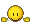Click Here To Show Diagram Code`[go]\$\$W Prevents liberty stealing throw-in\$\$ ---------------------------------------\$\$ | . . . . X . . . . . . . . . . . . . . |\$\$ | . X . . . X O . . . . . . . . . . . . |\$\$ | . . X X X O . O . O O O X X X X . . . |\$\$ | X X O O O O . . O X X X O O O X X . . |\$\$ | O O b . . . . . . O . X X O O O X . . |\$\$ | . a . O . . . O O X X X X X . O O X . |\$\$ | . . O O O X X O X X O X O O O O X X . |\$\$ | . . O . O O X O X . O X X X O X O . . |\$\$ | . X X O X O O X X X O O X O O X . . . |\$\$ | 3 O X , X X O X O X X O O O X X X X . |\$\$ | . O X X . O . O O X O . O X O O O . . |\$\$ | 4 O O X X O O O X O O O O X X X O . . |\$\$ | 2 c 5 . O X X X X X X O X X . . . . . |\$\$ | . 1 O O O O O O X X . X O X . . O . . |\$\$ | O O X B O X X X . X . X O . . . . . . |\$\$ | X X . X X . . O . , X . X X . O . . . |\$\$ | . . . . . . X . . X . . . X . . . . . |\$\$ | . . . . . . . O . . X . X . . . . . . |\$\$ | . . . . . . . . . . . X . . . . . . . |\$\$ ---------------------------------------[/go]`The solid connection,in this diagram, does not make two eyes, either, but it prevents the liberty stealing throw-in at that point.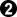-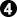holds White to one eye, but it is a big eye. Even with the dame at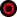filled, the big eye gives ample liberties for White to win the semeai. White can answer "a" at "b". Note that if Black triesat 5, White "c" makes two eyes. Click Here To Show Diagram Code`[go]\$\$W Easy life\$\$ ---------------------------------------\$\$ | . . . . X . . . . . . . . . . . . . . |\$\$ | . X . . . X O . . . . . . . . . . . . |\$\$ | . . X X X O . O . O O O X X X X . . . |\$\$ | X X O O O O . . O X X X O O O X X . . |\$\$ | O O . . . . . . . O . X X O O O X . . |\$\$ | . . . O . . . O O X X X X X . O O X . |\$\$ | . . O O O X X O X X O X O O O O X X . |\$\$ | . . O . O O X O X . O X X X O X O . . |\$\$ | . X X O X O O X X X O O X O O X . . . |\$\$ | 6 O X , X X O X O X X O O O X X X X . |\$\$ | 7 O X X . O . O O X O . O X O O O . . |\$\$ | . O O X X O O O X O O O O X X X O . . |\$\$ | 3 5 4 2 O X X X X X X O X X . . . . . |\$\$ | . 1 O O O O O O X X . X O X . . O . . |\$\$ | O O X . O X X X . X . X O . . . . . . |\$\$ | X X . X X . . O . , X . X X . O . . . |\$\$ | . . . . . . X . . X . . . X . . . . . |\$\$ | . . . . . . . O . . X . X . . . . . . |\$\$ | . . . . . . . . . . . X . . . . . . . |\$\$ ---------------------------------------[/go]`Note thatcan now be met with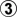, making a one point eye and living easily.

 Page 1 of 1 All times are UTC - 8 hours [ DST ] Powered by phpBB © 2000, 2002, 2005, 2007 phpBB Grouphttp://www.phpbb.com/